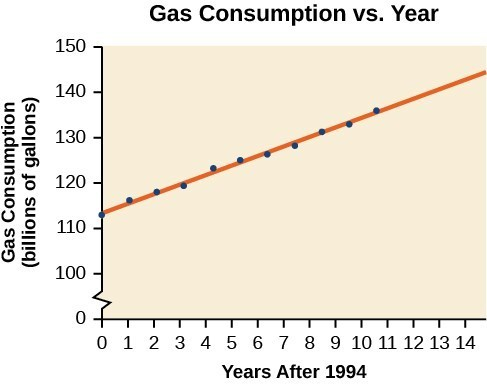## Use a linear model to make predictions

Once we determine that a set of data is linear using the correlation coefficient, we can use the regression line to make predictions. As we learned previously, a regression line is a line that is closest to the data in the scatter plot, which means that only one such line is a best fit for the data.

### Example 6: Using a Regression Line to Make Predictions

Gasoline consumption in the United States has been steadily increasing. Consumption data from 1994 to 2004 is shown in the table below. Determine whether the trend is linear, and if so, find a model for the data. Use the model to predict the consumption in 2008.

 Year ’94 ’95 ’96 ’97 ’98 ’99 ’00 ’01 ’02 ’03 ’04 Consumption (billions of gallons) 113 116 118 119 123 125 126 128 131 133 136

The scatter plot of the data, including the least squares regression line, is shown in Figure 8.Figure 8

### Solution

We can introduce new input variable, t, representing years since 1994.

The least squares regression equation is:

$C\left(t\right)=113.318+2.209t$

Using technology, the correlation coefficient was calculated to be 0.9965, suggesting a very strong increasing linear trend.

Using this to predict consumption in 2008 $\left(t=14\right)$,

$\begin{cases}C\left(14\right)=113.318+2.209\left(14\right)\hfill \\ \text{ }=144.244\hfill \end{cases}$

The model predicts 144.244 billion gallons of gasoline consumption in 2008.

### Try It 2

Use the model we created using technology in Example 6 to predict the gas consumption in 2011. Is this an interpolation or an extrapolation?

Solution